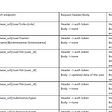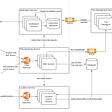# DS & Algo Problems — Binary Search and the Russian Doll

In the last post on binary search optimization, we found out that we can solve optimization problems using binary search under certain conditions. In this post we are going to look at another class of optimization problems that can again be solved using binary search technique. This class of problem is called the Longest Increasing Subsequence. I fondly call it the Russian Doll problem.

In its most simplest form, we are given an array A of size N. Each element of the array A is a real number. We need to find the length of the longest increasing subsequence in A.

One approach to find the length of longest increasing subsequence is to use dynamic programming. Let F[i] be the length of the longest increasing subsequence ending at index ‘i’. Then we can recursively find F[i] as follows:

F[i] = max(1 + F[j]) for all j < i s.t. A[j] < A[i]. If there is no j < i s.t. A[j] < A[i] then F[i] = 1.

The time complexity of the above approach is O(N²).

There is a better way to solve this problem.

Let F[k] be the smallest number ending for a k-length increasing subsequence. There could be many k-length increasing subsequences but F[k] represents only one having the smallest ending number (yes it is possible to have more than one k-length increasing subsequences ending with the same number).

Now at index ‘i’, we do binary search over ‘k’, because increasing ‘k’ will never decrease F[k]. Thus F[k] is a non-decreasing function of ‘k’. Also we search left half or right half based on whether F[k] < A[i]. If F[k] < A[i] then we have the liberty to increase ‘k’ else decrease ‘k’. If we are able to find some ‘k’ s.t. F[k] < A[i] and F[k+1] ≥ A[i] then it implies that the maximum length increasing subsequence ending at A[i] would be k+1, then we simply update F as follows:

F[k+1] = min(F[k+1], A[i])

Because we have found a (k+1)-length increasing subsequence ending at index ‘i’.

Also note that at any point in time the possible values of ‘k’ in F[k] is in the range [1, M] where M is the maximum length increasing subsequence found so far.

Time complexity of the above approach is O(N*logN).

This principle can be applied for any problem that requires finding a longest increasing subsequence. In general, given we have N, D-dimensional objects (length, breadth, width, …etc.), we can find the longest sequence of objects X such that we can put X[i] completely inside X[i+1] i.e. all dimensions of X[i] are smaller than X[i+1]. Think about the Matryoshka Russian dolls.

Let’s look at few problems to understand the concept better:

Problem 1:

You have a number of envelopes with widths and heights given as a pair of integers `(w, h)`. One envelope can fit into another if and only if both the width and height of one envelope is greater than the width and height of the other envelope.

What is the maximum number of envelopes can you put one inside other ?

Solution:

In order to solve this problem, we need to first convert this problem into a longest increasing subsequence problem. We can do this by either sorting the envelopes array ‘E’ on either ‘widths’ or ‘heights’. In that way the longest sequence of envelopes is a subsequence of the sorted array (because both width and height needs to be increasing).

After sorting on say ‘widths’ we find the longest increasing subsequence using the ‘heights’. Let H[k] be the smallest height ending for a k-length increasing subsequence. E[i] denotes the i-th envelope after sorting on ‘widths’ and E[i] is the height of the i-th envelope.

Now at index ‘i’, we do binary search over ‘k’, because increasing ‘k’ will never decrease H[k]. If we are able to find some ‘k’ s.t. H[k] < E[i] and H[k+1] ≥ E[i] then it implies that the maximum length increasing height subsequence ending at E[i] would be k+1, then we simply update H as follows:

H[k+1] = min(H[k+1], E[i])

Time complexity of the above approach is O(N*logN) where N is the number of envelopes.

What if there were multiple dimensions (x1, x2, x3, … xM) instead of just width and height. In that case, we would first sort on ‘x1’ to make the problem as a longest increasing subsequence, then would represent:

F[k][d] be the smallest value ending for a k-length increasing subsequence for the d-th dimension in the sorted array, where 2 ≤ d ≤ M. Then at index ‘i’, would search through all dimensions ‘d’. Let the maximum lengths discovered in each dimension be (k_2, k_3, …, k_M).

Maximum length of increasing subsequence ending at index ‘i’ is then = minimum(k_2, k_3, …, k_M) + 1. Time complexity of this approach is O(N*M*logN) where M is the number of dimensions.

Problem 2:

Given an array A consisting of N distinct integers, and another array B consisting of M integers (not necessarily distinct). You need to find the minimum number of elements to be added in B so that A becomes sub-sequence of B, we can add elements at any position in array B.

A subsequence is a sequence that car be derived by deleting some or no elements from the sequence without changing the order of the remaining elements.

Solution:

If one is familiar with concept of longest common subsequence, then they would be quick to observe that this problem requires you to find the length of the longest common subsequence between A and B, LCS(A, B) and then for minimum additions subtract length of LCS(A, B) from length of A.

LCS(A, B) takes O(N*M) time complexity to run.

Observing that the array A has all unique elements, we can compute the LCS(A, B) in O(M+N*logN).

For each element A[i] find the corresponding element in B and replace it with ‘i’. Discard all elements in B which are not present in A. For e.g. given the arrays A=[1,2,3,4,5] and B=[2,5,6,4,9,12]

Then the elements [2, 5, 4] in B are also present in A. Replace them with the corresponding index in A i.e. [1, 4, 3].

Then find the length of the longest increasing subsequence of the new array, which is 2 [1,4] or [1,3]. Since the length of A is 5, then we need to add 3 more elements to B to make A a subsequence of B. To make A a subsequence of B, we can add [2,3,5] to [1,4] or [2,4,5] to [1,3].

Time complexity of the above approach is O(M + N*logN) because we scan through the array B once to discard all the ‘non-A’ elements and then replace with the index of A. Longest increasing subsequence is computed on an array of length ≤ N.

Problem 3:

Tourist walks along the X axis. He can choose either of two directions and any speed not exceeding V. He can also stand without moving anywhere. He knows from newspapers that at time t1 in the point with coordinate x1 an interesting event will occur, at time t2 in the point with coordinate x2 — another one, and so on up to (xn, tn). Interesting events are short so we can assume they are immediate. Event i counts visited if at time ti tourist was at point with coordinate xi.

Write program tourist that will find maximum number of events tourist can visit.

Solution:

For any 2 events i and j, where ti ≤ tj, j is reachable from i if abs(xi-xj)/(tj-ti) ≤ V.

So if xi ≤ xj, then (xj-xi) ≤ (tj-ti)*V or ti*V-xi ≤ tj*V-xj. Since xi ≤ xj and also ti ≤ tj thus ti*V+xi ≤ tj*V+xj.

Similarly if xi ≥ xj, then (xi-xj) ≤ (tj-ti)*V or ti*V+xi ≤ tj*V+xj. Since xi ≥ xj and also ti ≤ tj thus ti*V-xi ≤ tj*V-xj.

Thus if ti*V-xi ≤ tj*V-xj and ti*V+xi ≤ tj*V+xj then event j is reachable from event i.

Let pi = ti*V-xi and qi = ti*V+xi, then instead of representing events with (xi, ti) we represent events as (pi, qi). Similar to the 2D Russian doll problem, instead of (width, height) we need to find the longest increasing subsequence such that pi ≤ pj and qi ≤ qj for i < j.

Thus first sort on ‘p’ values and then let f[k] denote the k-length increasing subsequence starting with maximum value of ‘q’. Since f[k] is an increasing function we can perform binary search on f[k] to find the maximum length subsequence starting from index ‘i’.

Time complexity of the approach is O(N*logN)

Problem 4 (The Pitfall):

Longest Common Increasing Subsequence — The sequence a1, a2, …, an is called increasing, if ai < ai + 1 for i < n.

The sequence s1, s2, …, sk is called the subsequence of the sequence a1, a2, …, an, if there exist such a set of indexes 1 ≤ i1 < i2 < … < ik ≤ n that aij = sj. In other words, the sequence s can be derived from the sequence a by crossing out some elements.

You are given two sequences of integer numbers. You are to find their longest common increasing subsequence, i.e. an increasing sequence of maximum length that is the subsequence of both sequences.

Solution:

Let the 2 sequences be denoted as ‘a’ and ‘b’. One approach to solve the problem is as follows:

For each index ‘i’ in the 1st sequence ‘a’, go through the entire 2nd sequence ‘b’. Let f[j][k] denote the minimum ending value for a k-length longest common increasing subsequence computed till index ‘j’ in sequence ‘b’.

If b[j] = a[i], then update f[j][k] similar to longest increasing subsequence. Do a binary search over ‘k’ and find the largest ‘k’ such that b[j] > f[j-1][k].

Then f[j][k+1] = min(f[j][k+1], f[j-1][k]+1)

If b[j] <> a[i], then f[j][k] = min(f[j][k], f[j-1][k]).

To obtain a possible longest common increasing subsequence, using a map ‘prev’ store the previous number in the sequence when a[i] = b[j] i.e. whenever for the highest possible value of ‘k’ we find b[j] > f[j-1][k], update prev[b[j]] = f[j-1][k].

Time complexity of the approach is O(N²logN).

But there is a better algorithm that solves the above in O(N²) time complexity without using the longest increasing subsequence strategy.

Let dp[j] store the length of LCIS ending at index ‘j’ in the sequence ‘b’.

‘current’ is the current length of LCIS ending with a[i]. Whenever we encounter b[j] < a[i] we update the value of ‘current’ to the length of LCIS ending at index ‘j’. Else if b[j] = a[i], we increment ‘current’ by 1.

Time complexity of the above approach is O(N²).

That’s all the problems for the time being. Hope you have understood concepts related to Longest Increasing Subsequence and Russian Doll.

In the next post, we are going to look at Data Structures for Sorted Sets which are a very handy set of data structures during interviews and programming contests.

--

--

--

## More from The Startup

Get smarter at building your thing. Follow to join The Startup’s +8 million monthly readers & +756K followers.

## Using the niddler IntelliJ plugin## Eight things you need to know about ISO 20022 XML Messages## Managing Group Projects## My Coding Journey — Day 1## Fleet management software: 7 factors to help you choose the right one## Hacking the Internal (Tryhackme)Engineer

## Custom Memory Allocator : malloc()## Ternary Search Convex Optimization